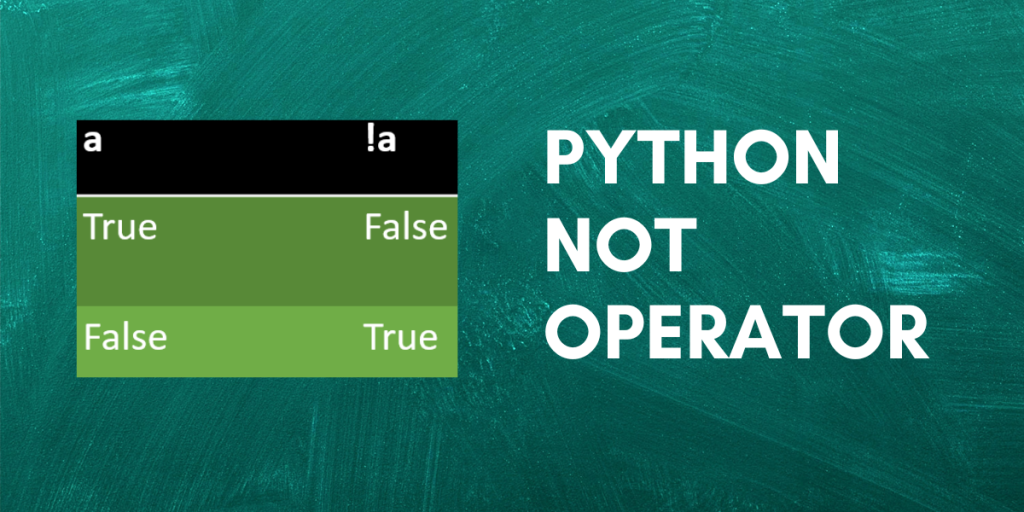# The ‘not’ Boolean Operator in PythonBoolean operators produce a single boolean output value from one or more input values. There are three boolean operators in boolean algebra: AND, OR, and NOT. Python uses `and`, `or`, and `not` to implement them. We shall learn about Python’s not operator in this tutorial.

## The not operator

It is used to get the negation of a value, i.e. it allows us to invert the truth value of a given boolean expression. This operator can be applied in boolean situations like if statements and while loops. It also functions in non-Boolean settings, enabling you to reverse the variables’ truth values.

The below table shows the outcomes for some input values when the not the operator is applied to them.

`not` is a unary operator which means it takes only one input value. It can be used with any boolean expression or Python object.

## Using not with conditional statements

Let’s see how the not operator in Python works with the different types of conditional statements we have.

### if statement

```num = 25

if not num%2==0:
print("num is an odd number")
else:
print("num is an even number")
```

Output:

```num is an odd number
```

Here, num%2 i.e. 25%2 equals 1 and not 0. The `if` statement checks whether num%2==0 is False. Since the condition is satisfied, the output says the number is odd. If it were an even number, the `else` condition would have been satisfied.

### while loop

```count = 1

while not count>5:
print(count)
count = count + 1
```

Output:

```1
2
3
4
5
```

The condition count>5 checks if the number of records is greater than 5. The `while` loop executes till `count` is less than 5. Once `count`‘s value becomes 6, the loop will terminate.

## Methods of implementing the not boolean operator in Python

### Method 1: Using the ‘not’ keyword

```var  = False
print(not var)
```

Output:

```True
```
```var = True
print(not var)
```

Output:

```False
```

Above are simple examples of using the `not` keyword in Python.

### Method 2: Using the ‘~’ operator

‘~’ is called the negation operator.

```flag = True

print("Flag is ", flag)

print("Flag is ", bool(~flag))
```

Output:

```Flag is  True
Flag is  False
```

In this example, the `flag` is set to `True` at first and its value is printed. In the next line, we first take the negation of `flag` by writing `~flag` and we have written `bool(~flag)` because if the flag was not a boolean at first, then it will be converted into a boolean.

### Method 3: Using the operator module

The operator module in Python provides various methods like addition, subtraction, exponentiation, left shift, right shift, etc. One among these many methods is `not_()`. It returns the negated value of the argument provided to it.

```import operator

initial_list = [False, True, True, False]
print("Initial list:", initial_list)
negated_list = []

for i in range(0, len(initial_list)):
negated_list.append(operator.not_(initial_list[i]))

print("Negated list:", negated_list)
```

Output:

```Initial list: [False, True, True, False]
Negated list: [True, False, False, True]
```

Here, we have first imported the operator module. Then we created a list of some boolean values. The loop iterates over the ‘initial_list’ and negates each value using the `not_()` method from the operator module and appends it to the ‘negated_list’.

### Method 4: Using the bitwise_not() method from NumPy

Numpy provides a `bitwise_not()` method to find the negation of a value. It performs bitwise NOT operation on its arguments.

```import numpy as np

print(np.bitwise_not(True))
print(np.bitwise_not(False))
```

Output:

```False
True
```

The above code uses the `bitwise_not()` method to negate only a single value. We can also use a NumPy array of elements as shown below.

```import numpy as np
a = np.array([True, False, False])
a = np.bitwise_not(a)
print(a)
```

Output:

```[False  True  True]
```

### Method 5: Using the invert() method from NumPy

This method also performs the bitwise NOT operation on arguments passed to it. It can be used on a single element or an array of elements.

```import numpy as np

print(np.invert(False))
print(np.invert(True))
```

Output:

```True
False```

Another example:

```import numpy as np

a = [True, False, False]
a = np.invert(a)
print(a)
```

Output:

```[False  True  True]
```

### Method 6: Using the logical_not() method from NumPy

This method can also be used for finding the negation of a boolean value. Just like the bitwise_not() and invert() methods, this method can also be applied to both, a single argument or a list of arguments as shown below.

```import numpy as np

print(np.logical_not(False))
print(np.logical_not(True))
```

Output:

```True
False
```

Another example:

```import numpy as np

a = [True, False, True]
a = np.logical_not(a)
print(a)
```

Output:

```[False  True False]
```

## Conclusion

That’s all! We have learnt about the not operator and also the different ways in which we can use it in Python.